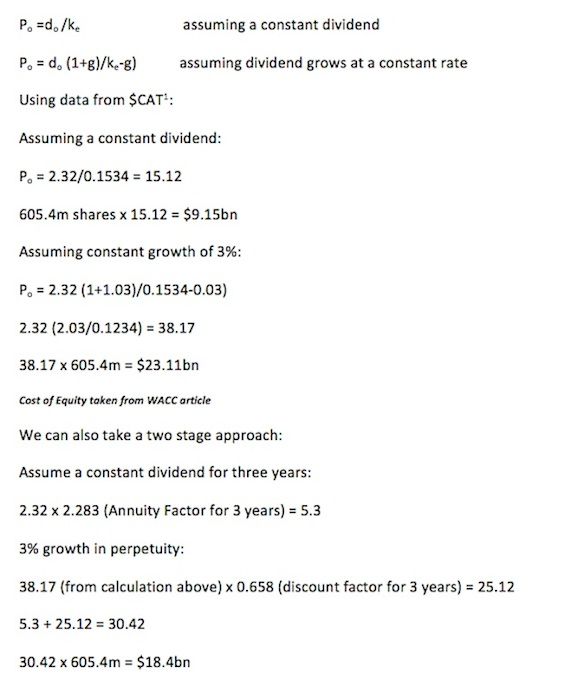# Using And Applying The Dividend Valuation ModelIn the final article of my series looking at ways in which to value a business, we will look at the Dividend Valuation Model (DVM). The theory is that an entity is worth the sum of its discounted future cash flows.

As noted in my previous article on discounted cash flows, if all free cash flows are paid out as dividends, and the same growth rate is used, the same value will be returned using the discounted cash flow method when the cost of equity is used to discount.

The model, as you know, is very straightforward but may be useful to investors to evaluate varying assumptions about an entity’s future prospects, perhaps as part of a scenario planning exercise. As with most methods, the assumptions are critical and the output will be a reflection of the information used in the calculation phase.

The formulae are:1. Data from Google Finance on \$CAT

One of the benefits of the Dividend Valuation Model is that we use dividends to value the company, which is a tangible return to the investor. It doesn’t give the total value of the entity though as debt is not included. Negative aspects are that we are making assumptions about future dividend growth, which is difficult given the life cycle of a business, and even more difficult if the entity has yet to pay a dividend. Looking at the formula, we can see if that the expected growth rate is greater than the cost of equity, the value of the entity will be infinite. This would cause valuation difficulties during periods of high growth for a business. Similarly if there has been an uneven growth rate, one may segment the growth periods and value them separately.

Finally, it is difficult to apply to unlisted companies as a proxy cost of equity would have to be used, as would assumptions on the dividend policy and growth rate. Having said that, the Dividend Valuation Model is another useful tool for the investor and when used in conjunction with the other methods we have considered, it at least allows a measured assessment to be undertaken.